# How to Concatenate Date and Text in Excel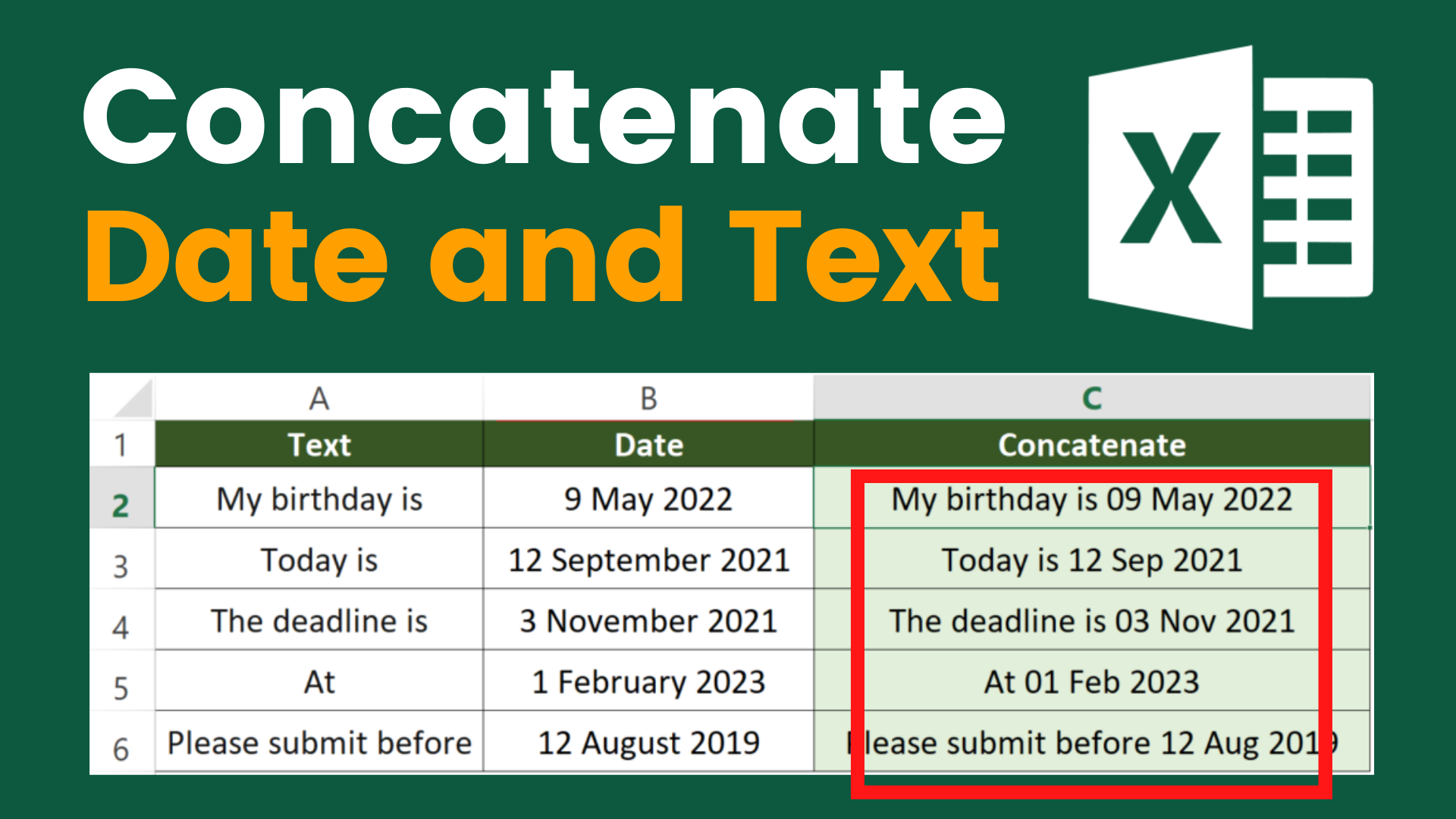Very often we need to concatenate date and text in Excel. However, this is not as intuitive as you may think. If you simply use the CONCATENATE function, you may end up having something like “44690”. This is because the way Excel see dates is different from how we see dates.

In this article, I will show you how to concatenate date and text in Excel in a correct date format. To help you better understand how to play around with date format in Excel, I put together a table where you can find all the date format expression.

If you have the following questions, I suggest you reading this until the end.

• How to concatenate date in Excel?
• How to concatenate date and text in Excel?
• How to concatenate date in Excel and receive a date?

You might also be interested in How to get value from every nth row?

## Example

In the above example, the concatenation of column A and B is in Column C. Next I will show you how to get the result by the formula. I will show you how to present the dates in different ways at the end of this article.

### What we would like to do

`To concatenate date and text`

You might also be interested in How to prevent duplicate entries in Excel?

## Concatenate date and text by TEXT function

### Formula in cell C2

`=A2&" "&TEXT(B2,"dd mmm yyyy")`

### How to use the formula

`=Text&Divider&TEXT(Date,"dd mmm yyyy")`

First you will need to use ampersand to concatenate the text and date. The only tricky part is we would apply a TEXT function here instead of simply putting the cell that contain the date. I will explain why we have to use TEXT function in the next part.

If you prefer to put the date ahead of the text, the formula would look like this

`=TEXT(Date,"dd mmm yyyy")&Text&Divider`

### How does this formula work

Here I will divide the formula into different part and explain it thoroughly.

TEXT function

`TEXT(Date,"dd mmm yyyy")`

TEXT function converts the value to text in a specific number format.

Excel stores dates as a sequential number instead of a date so that it can perform mathematical operations. Without using TEXT function to convert the sequential number into a date format, you will end up having a 5-digit number that starts with 4.

Here we are using TEXT to present the cell in a date format.

You might also be interested in How to Merge Cells Across Multiple Rows/Columns.

## Different way of presenting dates using TEXT function

Here I present almost all the possible date combinations. If you are not familiar with using the TEXT function yet, you can take a look at this and pick the one you are looking for.

### Formula in cell C3

`=A3&" "&TEXT(B3,"dd mmm")`

### Formula in cell C11

`=A11&" "&TEXT(B11,"dd mmm yy")`

### Formula in cell C15

`=A15&" "&TEXT(B15,"dd-mmm-yyyy")`

You might also be interested in How to Sum the Smallest N Values in Excel

You may want to understand a bit more about what the format is actually presenting.

Hungry for more useful Excel tips like this? Subscribe to our newsletter to make sure you won’t miss out on any of our posts and get exclusive Excel tips!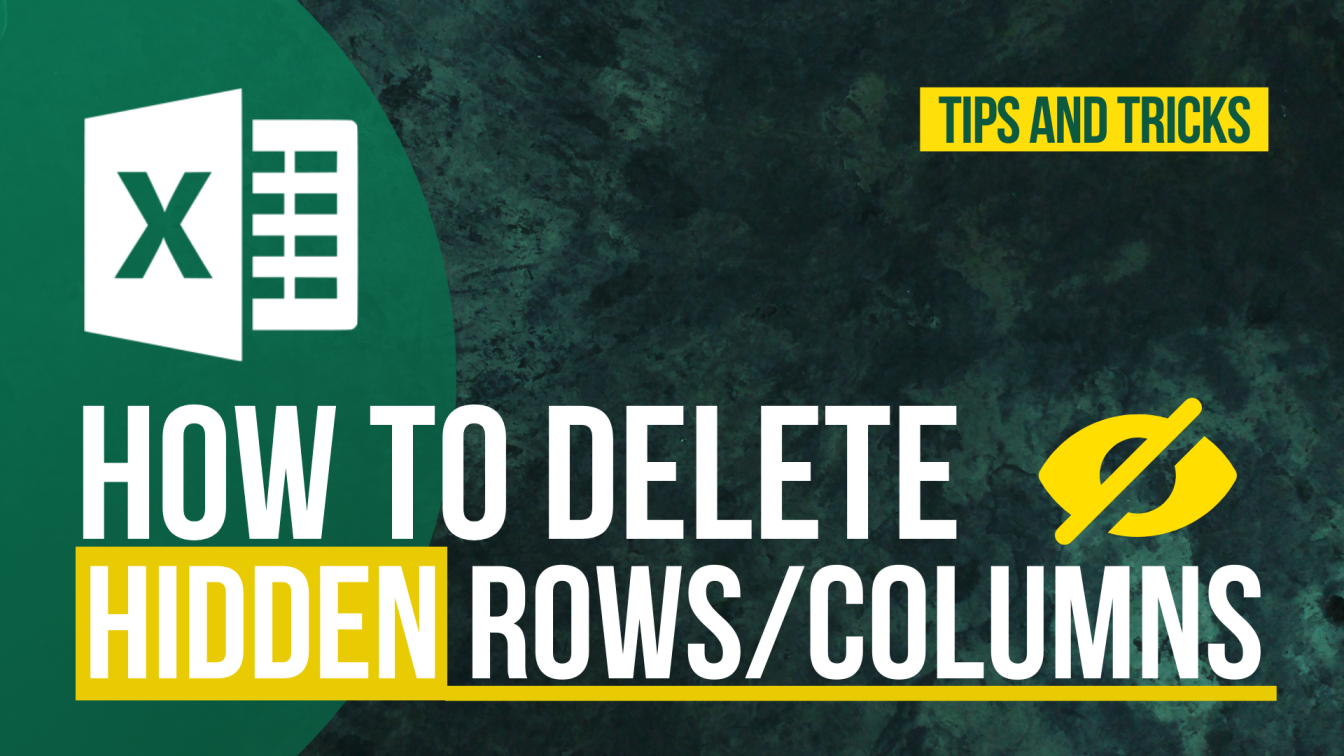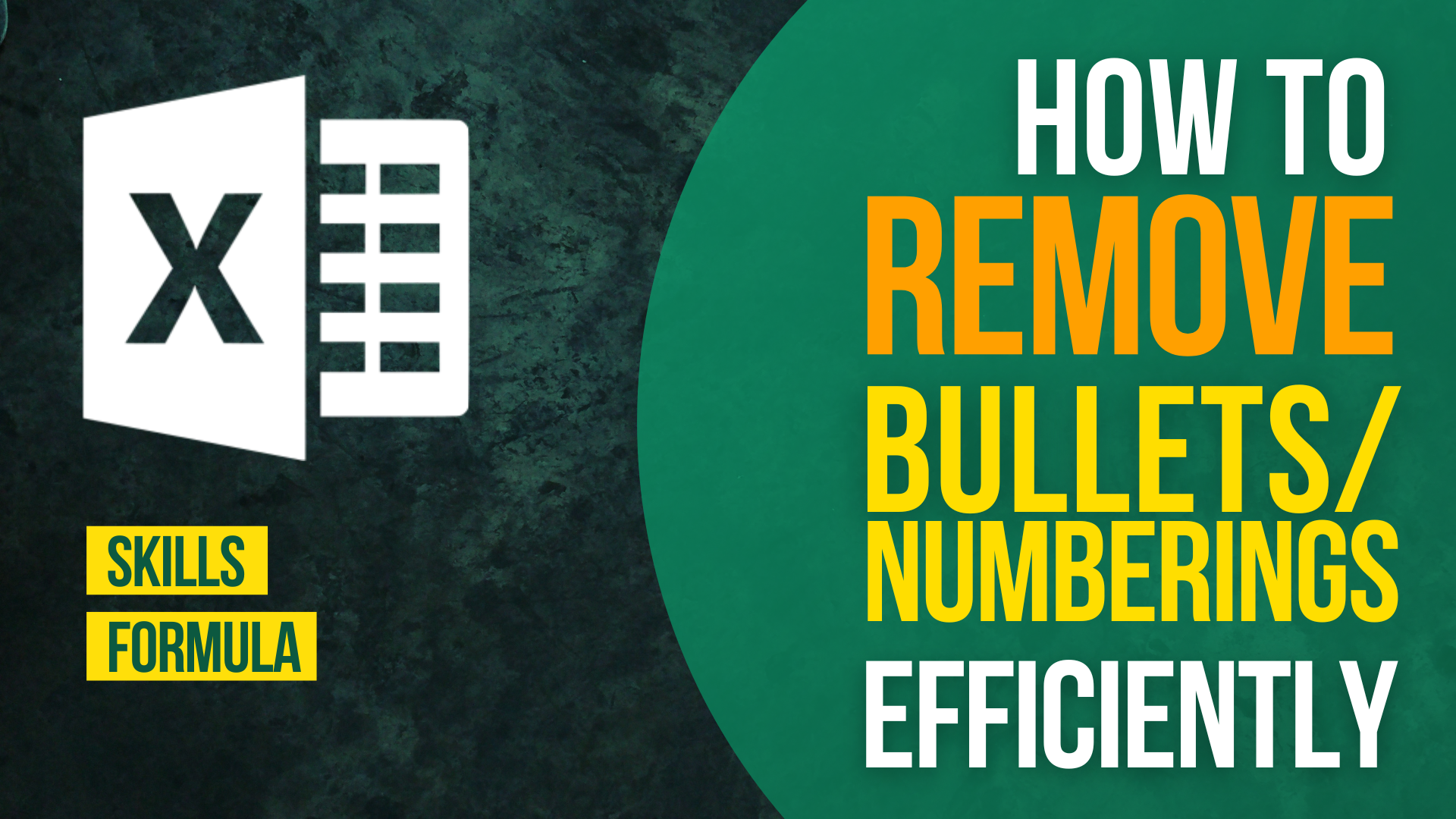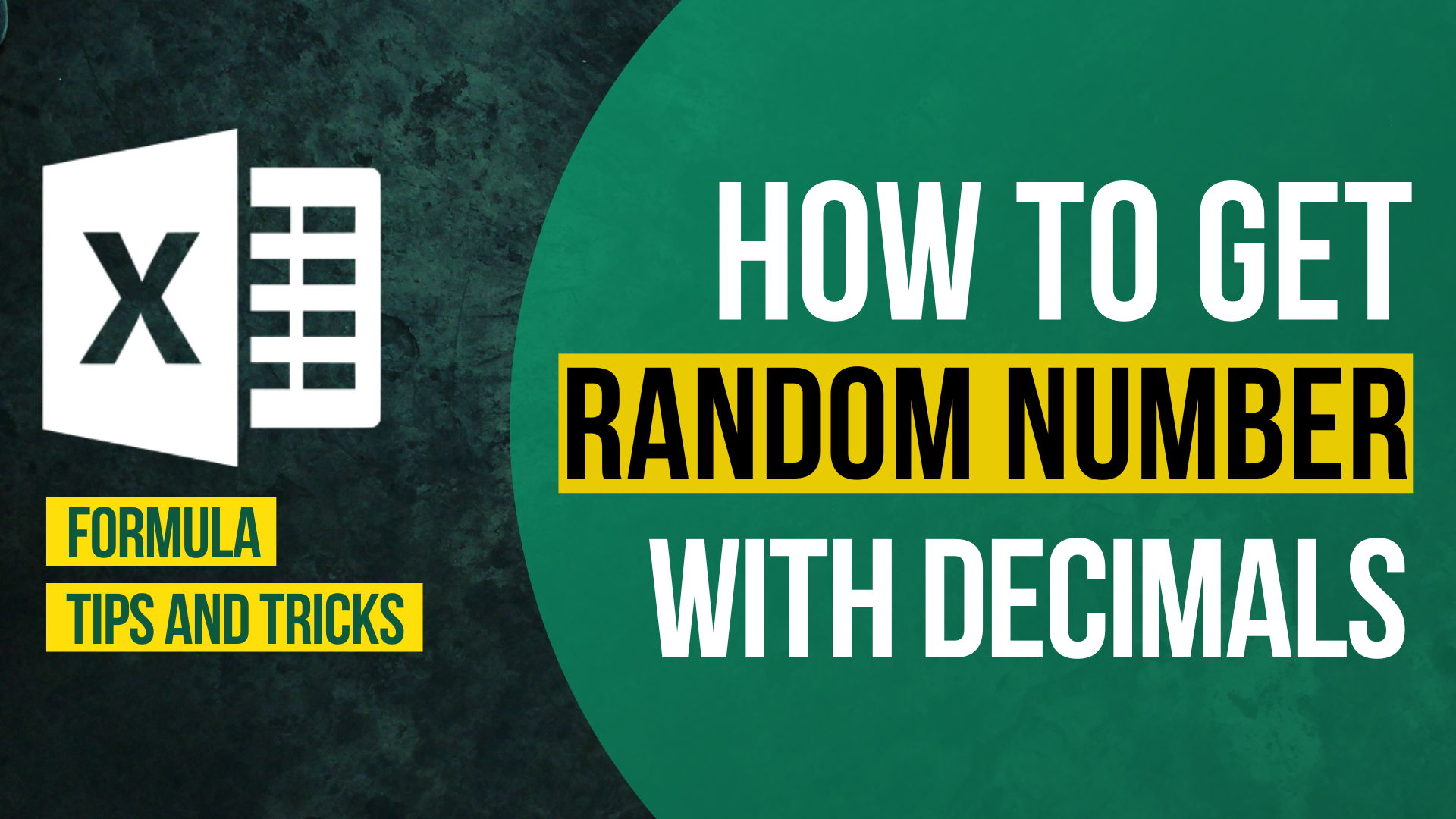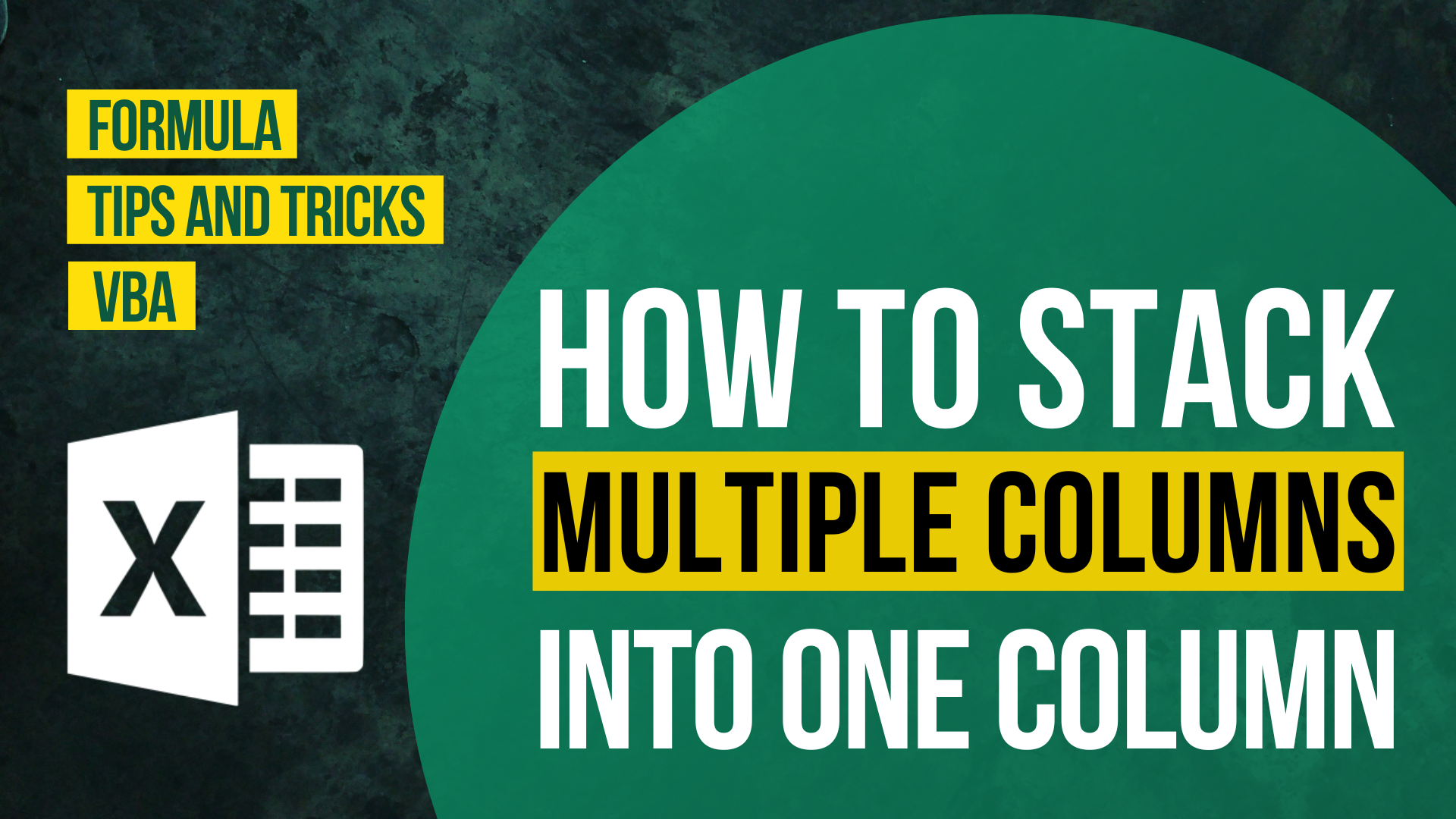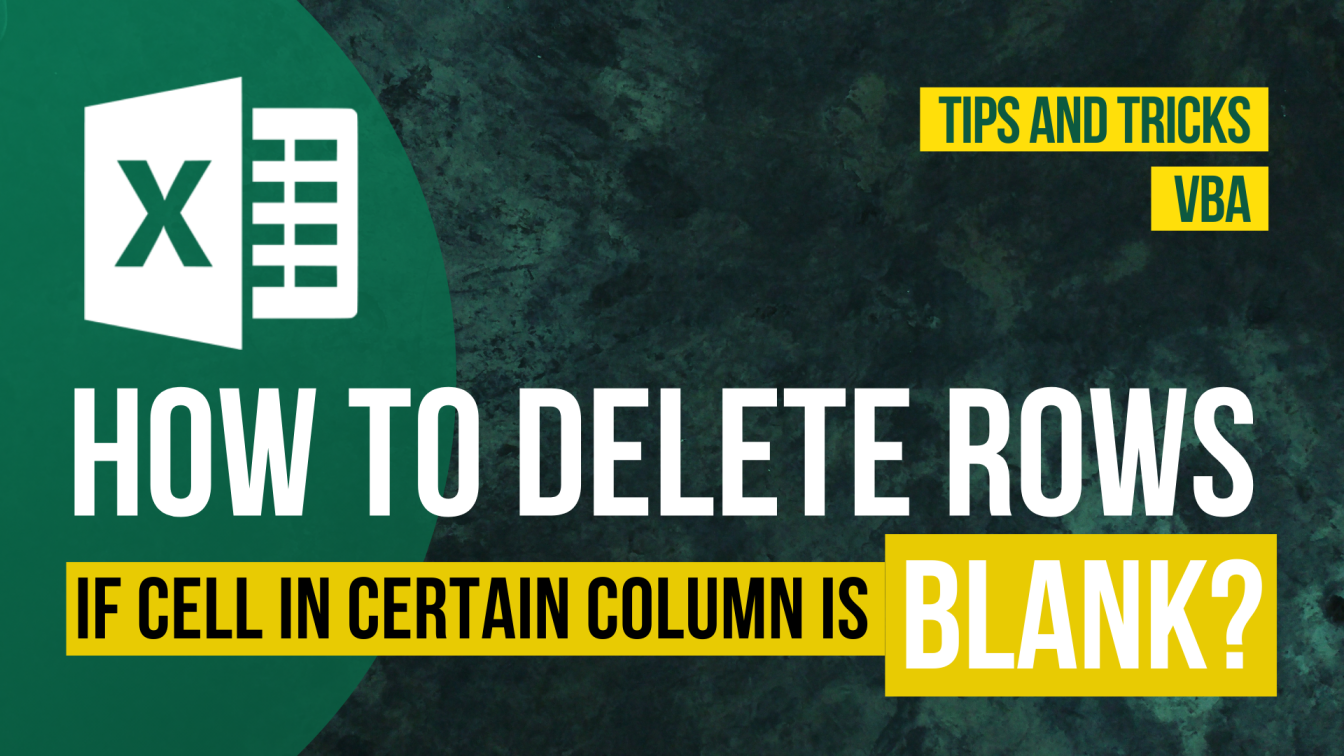How to Input Time Duration in Excel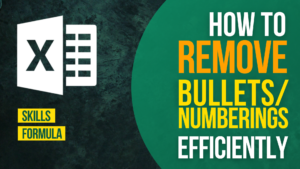How to Remove Bullets/Numberings in Excel

Popular posts